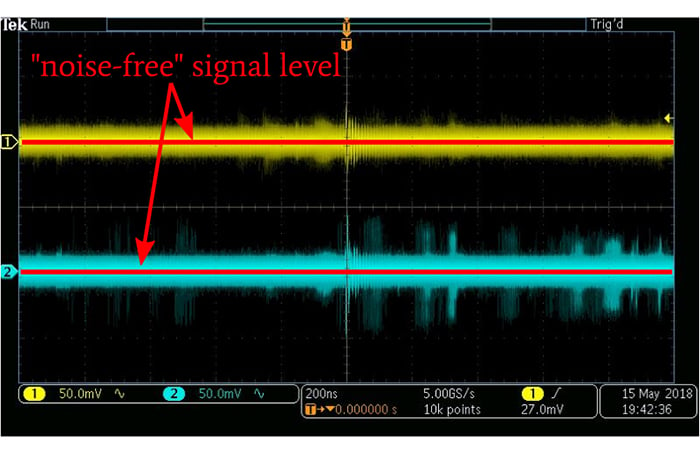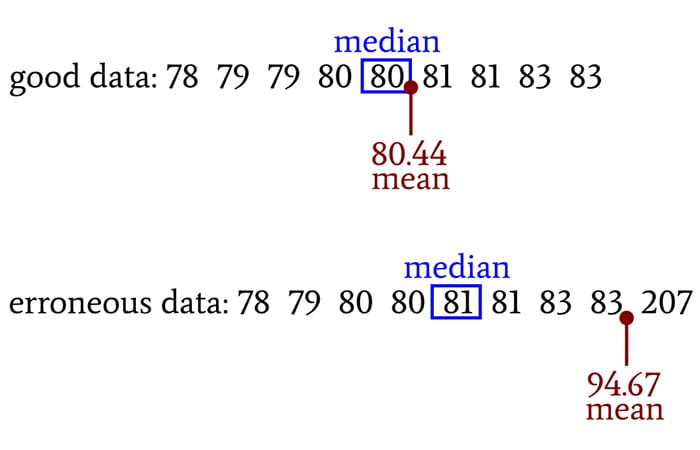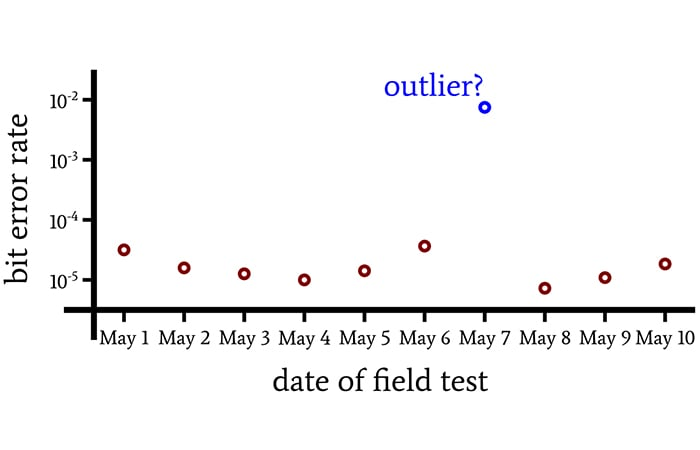Technical Article

# Descriptive Statistics in Electrical Engineering

June 22, 2020 by Robert Keim

## This article discusses statistical techniques that we can use to characterize an engineered system or analyze performance data.

In a previous article, I introduced the concept of statistical analysis and identified some prominent applications of statistical methods in the field of electrical engineering. In this article, we’ll explore statistical measures that belong to a category called descriptive statistics.

### The Importance of Descriptive Statistics

As the name suggests, descriptive statistics help us to describe data. If someone sends you a list consisting only of 172,800 numbers that appear to vary in random fashion, you are not likely to derive any useful information from those data. We can improve this situation by providing context; for example: “These are the two-sample-per-second temperatures that the system measured during the previous twenty-four hours.” Now you know the origin and significance of the data, but it’s awfully difficult to make overall assessments or achieve general understanding by inspecting a seemingly endless list of numbers.

This is why descriptive statistics are such valuable tools. They “summarize” potentially immense quantities of data by extracting salient characteristics and conveying general trends. In many cases, we can form judgments about a system or make decisions for future design activities based only on descriptive statistics—and it’s a good thing, because who would want to examine and evaluate 172,800 temperature measurements?

### Descriptive vs. Inferential Statistics

Descriptive statistics describe data. Inferential statistics, on the other hand, help us to make inferences about data. In ordinary language, an inference is an idea or opinion that someone forms by “reading between the lines,” i.e., by analyzing something and forming a conclusion that is suggested by, but not explicitly present in, the available information.

In statistics, we use mathematical procedures to infer meaningful relationships between variables. We might make the inference, based on data collected in the lab, that ambient temperatures between 45°C and 65°C make a microcontroller more susceptible to watchdog-timer resets. The data set itself does not contain this cause-and-effect relationship; the data set contains only numbers. The relationship between temperature and microcontroller behavior is a conclusion that we create by analyzing the numbers.

In my experience, inferential statistics are not particularly common in the design and characterization of electronic systems. They’re more prominent in research. Examples of inferential statistical techniques are regression analysis, t-tests, and analysis of variance (ANOVA).

### Basic Descriptive Statistics: Mean, Median, Mode

#### Mean

The statistical mean, also called the arithmetic mean, is one way of conveying the central tendency of a data set. To calculate a mean, you add all the values in the data set and divide by the number of values.

A mean is a straightforward way to reduce noise in a collection of measurements, because it approximates the value that would be observed if we eliminated the small positive and negative deviations caused by noise. We can also use the arithmetic mean to determine the DC offset of a waveform.#### Median

We can also use the median to convey central tendency. The median is the value that we observe if we take all the data points, line them up in ascending order, and select the datum that divides the data set into two portions of equal length. I find means more useful in engineering applications, but medians have an important advantage: a median is resistant to spurious values in the data set, whereas a mean can be severely altered by spurious values.

Let’s say we have an ambient light sensor that transmits eight-bit readings through a UART interface; it has produced nine illuminance measurements: 80, 81, 83, 79, 78, 81, 83, 80, 79 (the unit here is lux). The mean is 80.44 lux, and the median is 80 lux. In this case, both statistical measures give an accurate idea of the central tendency in the data.

However, suppose that a communication error caused the most significant bit of the last measurement to be interpreted as a one instead of a zero. This changes the value from 79 lux to 207 lux. Now, the mean is 94.67 lux, and the median is 81 lux. The central tendency conveyed by the mean has become seriously inaccurate, but the effect on the median is minor and would probably be insignificant in most applications.#### Outliers

Another way to deal with spurious values is to remove them from the data set before calculating the mean. In statistics, a spurious value is an outlier.

Statistical techniques can help us to identify outliers, but in general we cannot determine with certainty that a given datum is an outlier. There’s always an element of subjectivity, because a datum is a datum, and if we choose to apply the “outlier” label, we’re essentially saying to the datum, “Well, you don’t belong in this data set, despite the fact that you are indeed in the data set.”#### Mode

The value that occurs most frequently in a data set is the mode. If we’re thinking in terms of probability, the mode is the value that we are most likely to obtain when we randomly choose a datum from a data set.

Uses of statistical mode don’t arise frequently in the characterization of electronic systems, and as far as I know, it’s not prominent in digital signal processing either. Honestly, I don’t remember ever using statistical mode for any practical purpose.

### Conclusion

I hope that this has been a useful introduction to basic descriptive statistics, and that you clearly recognize the difference between descriptive statistics and inferential statistics. We’ll continue this topic in the next article, which discusses variance and standard deviation.• S
Steve W June 26, 2020

Thanks for this article - it is human nature to ignore basics when we have used terms like ‘median’ and ‘mean’ most of our lives.  Reading this article helped me understand how stats need to be properly used.  If you had asked me 20 minutes ago if median and mean meant the same thing, I’d have said “Yes”.  Steve W

Like.
•RK37 June 29, 2020
Thanks for the positive feedback, Steve. I'm glad that you enjoyed the article.
Like.
• H
herrmattoon July 04, 2020

Nice article. I am looking forward to the next ones about the variance and its square root ! Do you plan to tacle further stats topics ? Like identifying the nature of the noise on a signal based on its distribution ?

Like.
•RK37 July 10, 2020
Thanks for the positive feedback; I'm glad that you enjoyed this article. I've already written quite a few more articles on statistical topics. You should see those being gradually published over the next two or three weeks.
Like.